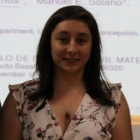﻿﻿ CI²MA - Publications | Undergraduate Thesis

# Undergraduate Thesis of Alejandra BarriosCareerMathematical Civil Engineering, Universidad de Concepción
Enrollment Year2011
Senior Year2018
Thesis TitleA discontinuous Galerkin method for the biharmonic problem

#### Thesis Summary:

A Hybridizable Discontinuous Galerkin (HDG) method for solving the biharmonic problem \$Delta^{2} u=f\$ is proposed and analyzed in this work. More precisely, we employ a Discontinuous Galerkin (DG) method based on a system of first-order equations, which we propose to approximate \$u\$, \$ abla u\$, \$mathcal{H}(u)\$ and \$ abla cdot mathcal{H}(u)\$ simultaneously, where \$mathcal{H}\$ corresponds to the Hessian matrix. This method allows us to eliminate all the interior variables locally to obtain a global system for \$hat{u}_{h}\$ and \$hat{boldsymbol{q}}_{h}\$ that approximate \$u\$ and \$ abla u\$, respectively, on the interfaces of the triangulation. As a consequence the only globally coupled degrees of freedom are those of the approximations of \$u\$ and \$ abla u\$ on the faces of the elements. We also carry out an priori error analysis using the orthogonal \$L^{2}\$-projection and conclude that the orders of convergence for the errors in the approximation of \$mathcal{H}(u)\$, \$ abla cdot mathcal{H}(u)\$, \$ abla u\$ and \$u\$ are \$k+1/2\$, \$k-1/2\$, \$k\$, and \$k+1\$, respectively, where \$k geq 1\$ is the polynomial degree of the discrete spaces. Our numerical results suggest that the approximations \$mathcal{H}(u)\$, \$ abla u\$ and \$u\$ converge with optimal order \$k+1\$ and the approximation of \$ abla cdot mathcal{H}(u)\$ converge with suboptimal order \$k\$.

Thesis Director(s) Manuel Solano
Thesis Project Approval Date2017, March 01
Thesis Defense Date2018, November 19
Professional Monitoring
PDF TesisDownload Thesis PDF(No publications)

<< Back to list of Undergraduate Thesis.

 CI²MA, CENTER FOR RESEARCH IN MATHEMATICAL ENGINEERING, UNIVERSIDAD DE CONCEPCIÓN - MAILBOX 160-C, CONCEPCIÓN, CHILE, PHONE: +56-41-2661324